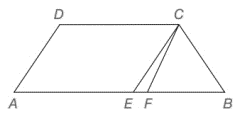Chapter 4.4, Problem 38EElementary Geometry For College St...

7th Edition
Alexander + 2 others
ISBN: 9781337614085

Solutions

Chapter
SectionElementary Geometry For College St...

7th Edition
Alexander + 2 others
ISBN: 9781337614085
Textbook Problem

Given: A B ¯ | | D C ¯ m ∠ A = m ∠ B = 56 ° C E ¯ | | D A ¯       and C F → bisects ∠ D C B Find: m ∠ F C ETo determine

To Find:

mFCE

Explanation

Consider the following diagram.

Given:

AB¯||DC¯, mA=mB=56°, CE¯||DA¯, CF bisects DCB.

Consider the trapezoid ABCD.

It is given that AB¯||DC¯ and mA=mB=56°

Still sussing out bartleby?

Check out a sample textbook solution.

See a sample solution

The Solution to Your Study Problems

Bartleby provides explanations to thousands of textbook problems written by our experts, many with advanced degrees!

Get Started

Explain what happens during each of the two stages of the two-factor ANOVA.

Essentials of Statistics for The Behavioral Sciences (MindTap Course List)

Through (4, 5), parallel to the y-axis

Single Variable Calculus: Early Transcendentals, Volume I

Solve for x: e2x1 = 10. a) 12(1+10e) b) 3 + ln 10 c) 2 + ln 10 d) 1+ln102

Study Guide for Stewart's Single Variable Calculus: Early Transcendentals, 8th